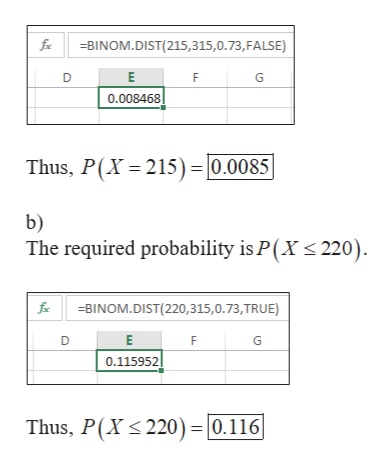# 13.) Seventy three percent of drivers carry jumper cables in their vehicles. For a random sample of 315 drivers, Binomial Distributions  (Show how you get these either by formula or calculator) a.) What is the probability that exactly 215 carry jumper cables in their vehicle?b.) What is the probability that at most 220 carry jumper cables in their vehicles?c.) What is the probability that more than 212 carry jumper cables in their vehicles?d.) What is the mean value of the number of drivers that carry jumper cables in their vehicle?e.) What is the standard deviation?

Question
13 views

13.) Seventy three percent of drivers carry jumper cables in their vehicles. For a random sample of 315 drivers, Binomial Distributions  (Show how you get these either by formula or calculator)

a.) What is the probability that exactly 215 carry jumper cables in their vehicle?

b.) What is the probability that at most 220 carry jumper cables in their vehicles?

c.) What is the probability that more than 212 carry jumper cables in their vehicles?

d.) What is the mean value of the number of drivers that carry jumper cables in their vehicle?

e.) What is the standard deviation?

check_circle

Step 1

The probability that a driver carry jumper cables in the vehicle (p) is 0.73.

Sample size (n) = 315

Let X be the number of drivers in the sample who carry jumper cables in their vehicles, which follows binomial distribution with n=315 and p=0.73.

As n is large, the required probabilities can be calculated using Excel function “BINOMDIST”.

Step 2

a)

The required probability is P(X = 215). The calculation is shown below:help_outlineImage Transcriptionclose-BINOM.DIST(215,315,0.73, FALSE) E G 0.008468 Thus, P(X 215) - |0.0085 b) The required probability is P(X 220) fx BINOM.DIST(220,315,0.73,TRUE) E E 0.115952 Thus, P(X 220 = 0.116 fullscreen
Step 3

c)

The required probability is P(...

### Want to see the full answer?

See Solution

#### Want to see this answer and more?

Solutions are written by subject experts who are available 24/7. Questions are typically answered within 1 hour.*

See Solution
*Response times may vary by subject and question.
Tagged in

### Other